# Euler identity

(diff) ← Older revision | Latest revision (diff) | Newer revision → (diff)

The relationwhereis an arbitrary real number and the product extends over all prime numbers. The Euler identity also holds for all complex numbers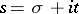with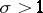.

The Euler identity can be generalized in the formwhich holds for every totally-multiplicative arithmetic function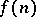for which the series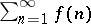is absolutely convergent.

Another generalization of the Euler identity is the formula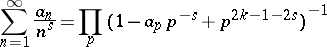for the Dirichlet seriescorresponding to the modular functions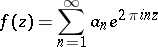of weight, which are the eigen functions of the Hecke operator.

How to Cite This Entry:
Euler identity. Encyclopedia of Mathematics. URL: http://encyclopediaofmath.org/index.php?title=Euler_identity&oldid=11612
This article was adapted from an original article by S.A. Stepanov (originator), which appeared in Encyclopedia of Mathematics - ISBN 1402006098. See original article